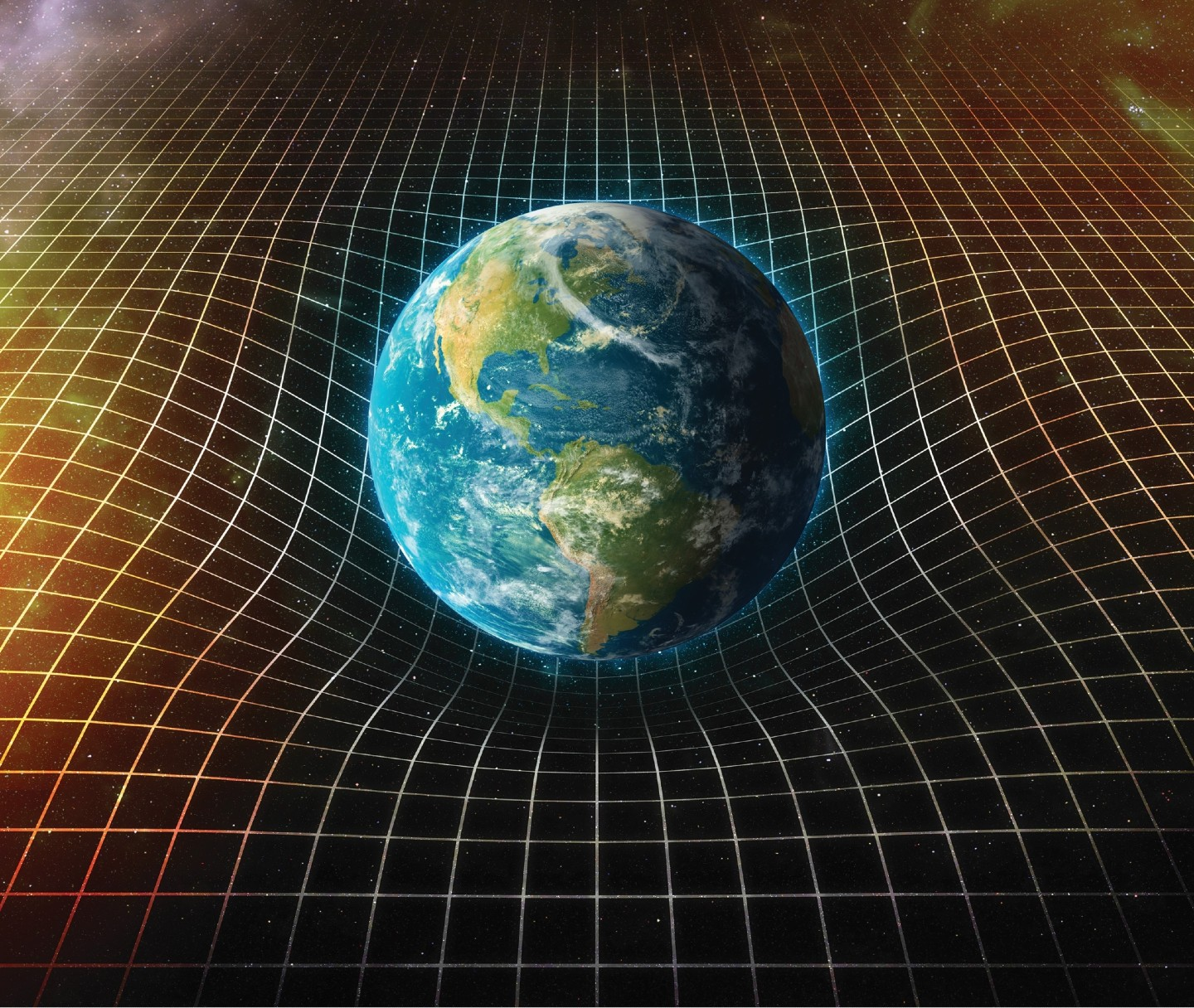## A Brief Overview of Gravity and Gravitational Force

### An introduction to Newton’s Law of Gravitation, specetime geometry, and quantum gravity.

#### Gravity

The word gravity has many definitions. As defined by dictionary.com, gravity means “the force of attraction by which terrestrial bodies tend to fall toward the center of the earth,” or “heaviness or weight,” or “serious or critical nature,” among other definitions (Dictionary.com, n.d.). In science courses, we mainly use the word gravity to describe the force rather than the gravity of the situation, unless, that is, there is a problem with our students’ understanding physical science!

#### Apples matter: Newton’s law of gravitation

Sir Issac Newton, the famous physicist, is generally credited with the discovery of gravity. In his seminal work published in 1687 titled Philosophiae Naturealis Principia Mathematica (Latin for “Mathematical Principles of Natural Philosophy”), generally referred to as The Principia (Jones, n.d.), Newton outlined his universal law of gravitation, which states:

“Every particle of matter in the universe attracts every other particle with a force that is directly proportional to the product of the masses of the particles and inversely proportional to the square of the distance between them.”

By watching apples fall from trees and understanding Earth’s influence on the moon, Newton concluded that apples and the moon were influenced by the same force, which he named gravity, from the Latin word gravitas, meaning “heaviness or weight.” He also wrote the following equation that puts the law stated above into symbols:

FG = G x [(m1m2)/(r2)]

Where:

• FG is the force of gravity (generally listed in the unit newton (N) where 1 N equals 1 kilogram (kg) times 1 meter (m) divided by the seconds (s) squared or, as symbols, 1 N = 1 kg×m×s-2)
• G is the gravitational constant and is equal to 6.674 x 10-11 N×m2×kg-2
• m1 and m2 are the masses of the 2 objects (in kg)
• r is the straight-line distance between the 2 objects (in m)

Force (FG) in the equation is always directed toward the other object, and according to Newton’s third law of motion, the force is always equal and opposite. Lighter objects with less mass (such as apples) fall to Earth (which is much larger than an apple) much faster than Earth falls toward them—even though the magnitude of the force is the same. Since the force (FG) is inversely proportional to the square of the distance between the objects, most of the time the distance between two objects limits the force of gravity, and only large objects with large masses have significant gravitational effects. In other words, we stick to Earth and do not fly away into space because Earth has a large mass that attracts us to it, not because we ate a big lunch.

#### Einstein: Gravity is a property of spacetime geometry

In 1915, Albert Einstein proposed that gravity itself is a property of spacetime geometry rather than a unique force as proposed by Newton. Key to Einstein’s theory, known as the theory of general relativity, is that the masses of objects themselves cause space to curve such that smaller objects become entangled, so to speak, in the warp in spacetime created by larger objects. Over time, experimental data, such as the bending of light from stars as confirmed by Arthur Eddington’s star photographs in 1919 (The Physics of the Universe, 2009) and changes in time records from satellites, have provided evidence that the theory has merit.

For example, satellites that are a part of the Global Positioning System (GPS) orbit Earth at an altitude of approximately 26,600 km (15,960 miles) and move at 14,000 km/h relative to Earth’s surface. Since the curvature of spacetime is less the further we move from the center of Earth (the center of its gravitational mass), clocks on satellites tick faster than identical clocks on the ground by roughly 38 microseconds per day. Stated another way, the weaker the gravitational potential (the further a clock is from the center of the mass of the source of gravitation), the faster time passes. In today’s world, the engineers and physical scientists designing GPS systems must take these relativistic effects into account, otherwise, over time, navigational systems that use GPS would not work correctly at Earth’s surface for ships, airplanes, etc.

#### Quantum Gravity: The folding of spacetime and gravity

The application of Einstein’s work does not stop with satellites. Today, physicists are trying to reconcile differences in the theories of gravity proposed by Newton and Einstein in the field of physics known as quantum gravity (QG), which seeks to explain gravity and curvatures of spacetime using past theories and quantum mechanics. The jury is out as to whether a single theory of gravity can capture all of the nuances because, at present, theoretical physicists are having difficulty reconciling differences in how time is treated in the two theories (Quanta Magazine, n.d.).

Hopefully, physicists will learn more in the future regarding the folding of spacetime and gravity. Since the laws of physics do not prevent the teleportation of large objects, maybe one day, instead of travelling from place to place in a car, airplane, or other means of transportation, we can just beam ourselves to a desired vacation location using our knowledge of gravity and the folding of spacetime! Until then, we keep learning about the physics of our universe.